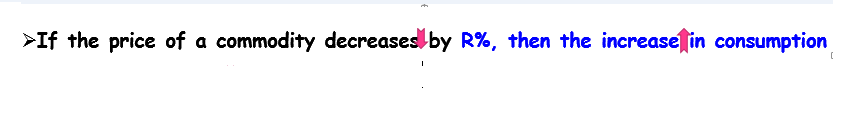# Percentage Quantitative Aptitude Interview Questions and Answers Part 1

## IMPORTANT FORMULAS

1. CONCEPT OF PERCENTAGE:

• By a certain percent, we mean that many hundredths. Thus, ”x” percent means x hundredths, written as x%.

2. PERCENTAGE INCREASE/DECREASE:3. RESULTS ON POPULATION:

• Let the population of a town be “P” now and suppose it increases at the rate of R% per annum.

4. RESULTS ON DEPRECIATION

• Let the present value of a machine be P. Suppose it depreciates at the rate of R% per annum.

1. A batsman scored 110 runs which included 3 boundaries and 8 sixes. What percent of his total score did he make by running between the wickets?